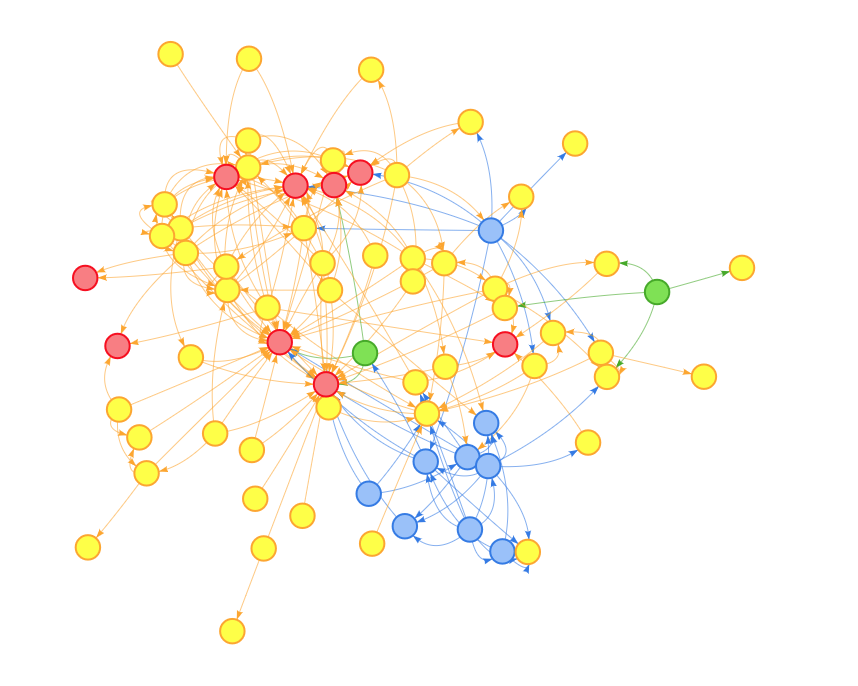We’re pleased to announce that sparklyr 0.8 is now available on CRAN! Sparklyr provides an R interface to Apache Spark. It supports dplyr syntax for working with Spark DataFrames and exposes the full range of machine learning algorithms available in Spark ML. You can also learn more about Apache Spark and sparklyr at spark.rstudio.com and the sparklyr webinar series. In this version, we added support for Spark 2.3, Livy 0.5, and various enhancements and bugfixes. For this post, we’d like to highlight a new feature from Spark 2.3 and introduce the mleap and graphframes extensions.

## Parallel Cross-Validation

Spark 2.3 supports parallelism in hyperparameter tuning. In other words, instead of training each model specification serially, you can now train them in parallel. This can be enabled by setting the parallelism parameter in ml_cross_validator() or ml_train_split_validation(). Here’s an example:

library(sparklyr)
sc <- spark_connect(master = "local", version = "2.3.0")
iris_tbl <- sdf_copy_to(sc, iris)

# Define the pipeline
labels <- c("setosa", "versicolor", "virginica")
pipeline <- ml_pipeline(sc) %>%
ft_vector_assembler(
c("Sepal_Width", "Sepal_Length", "Petal_Width", "Petal_Length"),
"features"
) %>%
ft_string_indexer_model("Species", "label", labels = labels) %>%
ml_logistic_regression()

# Specify hyperparameter grid
grid <- list(
logistic = list(
elastic_net_param = c(0.25, 0.75),
reg_param = c(1e-3, 1e-4)
)
)

# Create the cross validator object
cv <- ml_cross_validator(
sc, estimator = pipeline, estimator_param_maps = grid,
evaluator = ml_multiclass_classification_evaluator(sc),
num_folds = 3, parallelism = 4
)

# Train the models
cv_model <- ml_fit(cv, iris_tbl)


Once the models are trained, you can inspect the performance results by using the newly available helper function ml_validation_metrics():

ml_validation_metrics(cv_model)
spark_disconnect(sc)


## Pipelines in Production

Earlier this year, we announced support for ML Pipelines in sparklyr, and discussed how one can persist models onto disk. While that workflow is appropriate for batch scoring of large datasets, we also wanted to enable real-time, low-latency scoring using pipelines developed with sparklyr. To enable this, we’ve developed the mleap package, available on CRAN, which provides an interface to the MLeap open source project.

MLeap allows you to use your Spark pipelines in any Java-enabled device or service. This works by serializing Spark pipelines which can later be loaded into the Java Virtual Machine (JVM) for scoring without requiring a Spark cluster. This means that software engineers can take Spark pipelines exported with sparklyr and easily embed them in web, desktop or mobile applications.

To get started, simply grab the package from CRAN and install the necessary dependencies:

install.packages("mleap")
library(mleap)
install_maven()
install_mleap()

library(mleap)


Then, build a pipeline as usual:

library(sparklyr)
sc <- spark_connect(master = "local", version = "2.2.0")
mtcars_tbl <- sdf_copy_to(sc, mtcars)

# Create a pipeline and fit it
pipeline <- ml_pipeline(sc) %>%
ft_binarizer("hp", "big_hp", threshold = 100) %>%
ft_vector_assembler(c("big_hp", "wt", "qsec"), "features") %>%
ml_gbt_regressor(label_col = "mpg")
pipeline_model <- ml_fit(pipeline, mtcars_tbl)


Once we have the pipeline model, we can export it via ml_write_bundle():

# Export model
model_path <- file.path(tempdir(), "mtcars_model.zip")
transformed_tbl <- ml_transform(pipeline_model, mtcars_tbl)
ml_write_bundle(pipeline_model, transformed_tbl, model_path)
spark_disconnect(sc)


At this point, we’re ready to use mtcars_model.zip in other applications. Notice that the following code does not require Spark:

# Import model
model <- mleap_load_bundle(model_path)

# Create a data frame to be scored
newdata <- tibble::tribble(
~qsec, ~hp, ~wt,
16.2,  101, 2.68,
18.1,  99,  3.08
)

# Transform the data frame
transformed_df <- mleap_transform(model, newdata)
dplyr::glimpse(transformed_df)


Notice that MLeap requires Spark 2.0 to 2.3. You can find additional details in the production pipelines guide.

## Graph Analysis

The other extension we’d like to highlight is graphframes, which provides an interface to the GraphFrames Spark package. GraphFrames allows us to run graph algorithms at scale using a DataFrame-based API.

Let’s see graphframes in action through a quick example, where we analyze the relationships among package on CRAN.

library(graphframes)
library(dplyr)
sc <- spark_connect(master = "local", version = "2.1.0")

# Grab list of CRAN packages and their dependencies
available_packages <- available.packages(
contrib.url("https://cloud.r-project.org/")
) %>%
[(, c("Package", "Depends", "Imports")) %>%
as_tibble() %>%
transmute(
package = Package,
dependencies = paste(Depends, Imports, sep = ",") %>%
gsub("\\n|\\s+", "", .)
)

# Copy data to Spark
packages_tbl <- sdf_copy_to(sc, available_packages, overwrite = TRUE)

# Create a tidy table of dependencies, which define the edges of our graph
edges_tbl <- packages_tbl %>%
mutate(
dependencies = dependencies %>%
regexp_replace("\\\$$([^)]+)\\\$$", "")
) %>%
ft_regex_tokenizer(
"dependencies", "dependencies_vector",
pattern = "(\\s+)?,(\\s+)?", to_lower_case = FALSE
) %>%
transmute(
src = package,
dst = explode(dependencies_vector)
) %>%
filter(!dst %in% c("R", "NA"))


Once we have an edges table, we can easily create a GraphFrame object by calling gf_graphframe() and running PageRank:

# Create a GraphFrame object
g <- gf_graphframe(edges = edges_tbl)

# Run the PageRank algorithm
pagerank <- gf_pagerank(g, tol = 0.01)

pagerank %>%
gf_vertices() %>%
arrange(desc(pagerank))


We can also collect a sample of the graph locally for visualization:

library(gh)
library(visNetwork)
list_repos <- function(username) {
gh("/users/:username/repos", username = username) %>%
vapply("[[", "", "name")
}
rlib_repos <- list_repos("r-lib")
tidyverse_repos <- list_repos("tidyverse")
base_packages <- installed.packages() %>%
as_tibble() %>%
filter(Priority == "base") %>%
pull(Package)

top_packages <- pagerank %>%
gf_vertices() %>%
arrange(desc(pagerank)) %>%
head(75) %>%
pull(id)

edges_local <- g %>%
gf_edges() %>%
filter(src %in% !!top_packages && dst %in% !!top_packages) %>%
rename(from = src, to = dst) %>%
collect()

vertices_local <- g %>%
gf_vertices() %>%
filter(id %in% top_packages) %>%
mutate(
group = case_when(
id %in% !!rlib_repos ~ "r-lib",
id %in% !!tidyverse_repos ~ "tidyverse",
id %in% !!base_packages ~ "base",
TRUE ~ "other"
),
title = id) %>%
collect()

visNetwork(vertices_local, edges_local, width = "100%") %>%
visEdges(arrows = "to")

spark_disconnect(sc)Notice that GraphFrames currently supports Spark 2.0 and 2.1. You can find additional details in the graph analysis guide.